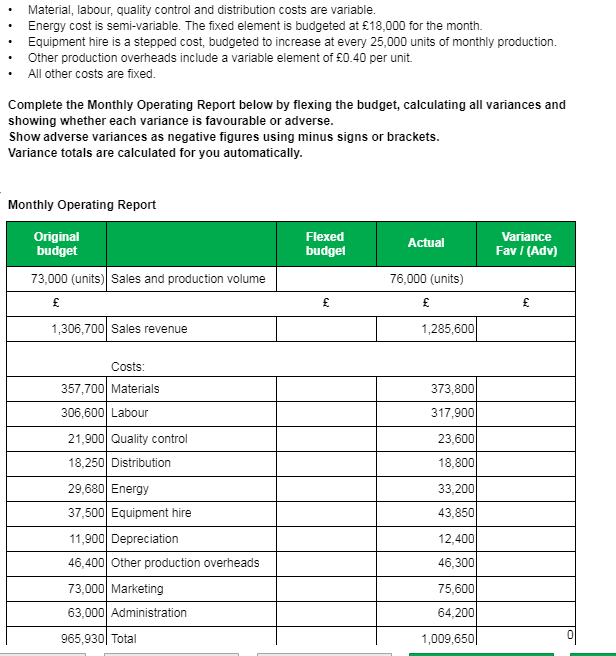# Monthly Operating Report

Hi,

I can work out all the other answer about from equipment hire and other production costs, please can someone help me out?

Equipment hire is - 50000
Other production cost answer is - 47600

Thanks

Sophie• For the production overheads you will need to apply the high low method to find what the fixed element is
OR simply 0.40*73000units = 29200 variable, therefore fixed is 46400-29200=17200
76000*0.40=30400 variable + fixed of 17200=47600

Equipment hire will be 73000/25000 units = 2.92 or 3 steps
£37500/3=£12500 = 1 step
therefore 76000/25000=3.04 or 4 x £12500 = £50000
AAT Level 2. 2018 Distinction
AAT Level 3. 2018 Distinction
AAT Level 4. 2019 FSLC 100% MABU 93% MADC 82% PDSY 80%
• dumutroba said:

For the production overheads you will need to apply the high low method to find what the fixed element is
OR simply 0.40*73000units = 29200 variable, therefore fixed is 46400-29200=17200
76000*0.40=30400 variable + fixed of 17200=47600

Equipment hire will be 73000/25000 units = 2.92 or 3 steps
£37500/3=£12500 = 1 step
therefore 76000/25000=3.04 or 4 x £12500 = £50000

@dumutroba thank you for helping, one question i do have is am i suppose to round for example where you put 2.92 or 3 and 3.04 or 4 do we automatically just round up as it isn't stated in the question what they want me to do?
• Yes you round up, because you are past the threshold, that being a step
AAT Level 2. 2018 Distinction
AAT Level 3. 2018 Distinction
AAT Level 4. 2019 FSLC 100% MABU 93% MADC 82% PDSY 80%